JMSLTM Numerical Library 7.2.0
com.imsl.stat

Class ANOVA

• Field Detail

• BONFERRONI

public static final int BONFERRONI
The Bonferroni method
Constant Field Values
• DUNN_SIDAK

public static final int DUNN_SIDAK
The Dunn-Sidak method
Constant Field Values
• ONE_AT_A_TIME

public static final int ONE_AT_A_TIME
The One-at-a-Time (Fisher's LSD) method
Constant Field Values
• TUKEY_KRAMER

public static final int TUKEY_KRAMER
The Tukey-Kramer method
Constant Field Values
• Constructor Detail

• ANOVA

public ANOVA(double[][] y)
/** Analyzes a one-way classification model.
Parameters:
y - is a two-dimension double array containing the responses. The rows in y correspond to observation groups. Each row of y can contain a different number of observations.
• ANOVA

public ANOVA(double dfr,
double ssr,
double dfe,
double sse,
double gmean)
Construct an analysis of variance table and related statistics. Intended for use by the LinearRegression class.
Parameters:
dfr - a double scalar value representing the degrees of freedom for model.
ssr - a double scalar value representing the sum of squares for model.
dfe - a double scalar value representing the degrees of freedom for error.
sse - a double scalar value representing the sum of squares for error.
gmean - a double scalar value representing the grand mean. If the grand mean is not known it may be set to not-a-number.
• Method Detail

Returns the adjusted R-squared (in percent).
Returns:
a double scalar value representing the adjusted R-squared (in percent)
• getArray

public double[] getArray()
Returns the ANOVA values as an array.
Returns:
a double array containing the following values:

 index Value 0 Degrees of freedom for model 1 Degrees of freedom for error 2 Total degrees of freedom 3 Sum of squares for model 4 Sum of squares for error 5 Total sum of squares 6 Model mean square 7 Error mean square 8 F statistic 9 p-value 10 R-squared (in percent) 11 Adjusted R-squared (in percent) 12 Estimated standard deviation of the model error 13 Mean of the response (dependent variable) 14 Coefficient of variation (in percent)

• getCoefficientOfVariation

public double getCoefficientOfVariation()
Returns the coefficient of variation (in percent).
Returns:
a double scalar value representing the coefficient of variation (in percent)
• getConfidenceInterval

public double[] getConfidenceInterval(double conLevel,
int i,
int j,
int compMethod)
Computes the confidence interval associated with the difference of means between two groups using a specified method.

getConfidenceInterval computes the simultaneous confidence interval on the pairwise comparison of means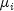and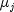in the one-way analysis of variance model. Any of several methods can be chosen. A good review of these methods is given by Stoline (1981). Also the methods are discussed in many elementary statistics texts, e.g., Kirk (1982, pages 114-127). Let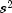be the estimated variance of a single observation. Letbe the degrees of freedom associated with. Let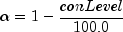The methods are summarized as follows:

Tukey method: The Tukey method gives the narrowest simultaneous confidence intervals for the pairwise differences of means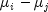in balanced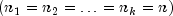one-way designs. The method is exact and uses the Studentized range distribution. The formula for the differenceis given by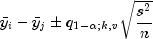where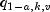is the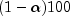percentage point of the Studentized range distribution with parametersand. If the group sizes are unequal, the Tukey-Kramer method is used instead.

Tukey-Kramer method: The Tukey-Kramer method is an approximate extension of the Tukey method for the unbalanced case. (The method simplifies to the Tukey method for the balanced case.) The method always produces confidence intervals narrower than the Dunn-Sidak and Bonferroni methods. Hayter (1984) proved that the method is conservative, i.e., the method guarantees a confidence coverage of at least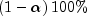. Hayter's proof gave further support to earlier recommendations for its use (Stoline 1981). (Methods that are currently better are restricted to special cases and only offer improvement in severely unbalanced cases, see, e.g., Spurrier and Isham 1985). The formula for the differenceis given by the following: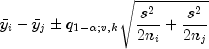Dunn-Sidak method: The Dunn-Sidak method is a conservative method. The method gives wider intervals than the Tukey-Kramer method. (For largeand smalland k, the difference is only slight.) The method is slightly better than the Bonferroni method and is based on an improved Bonferroni (multiplicative) inequality (Miller, pages 101, 254-255). The method uses the t distribution. The formula for the differenceis given by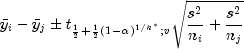where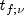is the 100f percentage point of the t distribution withdegrees of freedom.

Bonferroni method: The Bonferroni method is a conservative method based on the Bonferroni (additive) inequality (Miller, page 8). The method uses the t distribution. The formula for the differenceis given by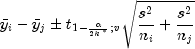Scheffé method: The Scheffé method is an overly conservative method for simultaneous confidence intervals on pairwise difference of means. The method is applicable for simultaneous confidence intervals on all contrasts, i.e., all linear combinations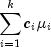where the following is true: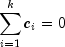The method can be recommended here only if a large number of confidence intervals on contrasts in addition to the pairwise differences of means are to be constructed. The method uses the F distribution. The formula for the differenceis given by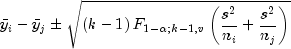where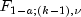is the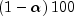percentage point of the F distribution with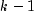anddegrees of freedom.

One-at-a-time t method (Fisher's LSD): The one-at-a-time t method is the method appropriate for constructing a single confidence interval. The confidence percentage input is appropriate for one interval at a time. The method has been used widely in conjunction with the overall test of the null hypothesis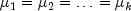by the use of the F statistic. Fisher's LSD (least significant difference) test is a two-stage test that proceeds to make pairwise comparisons of means only if the overall F test is significant. Milliken and Johnson (1984, page 31) recommend LSD comparisons after a significant F only if the number of comparisons is small and the comparisons were planned prior to the analysis. If many unplanned comparisons are made, they recommend Scheffe's method. If the F test is insignificant, a few planned comparisons for differences in means can still be performed by using either Tukey, Tukey-Kramer, Dunn-Sidak or Bonferroni methods. Because the F test is insignificant, Scheffe's method will not yield any significant differences. The formula for the differenceis given by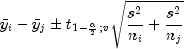Parameters:
conLevel - a double specifying the confidence level for simultaneous interval estimation. If the Tukey method for computing the confidence intervals on the pairwise difference of means is to be used, conLevel must be in the range [90.0, 99.0]. Otherwise, conLevel must be in the range
[0.0, 100.0). One normally sets this value to 95.0.
i - is an int indicating the i-th member of the pair difference,. i must be a valid group index.
j - is an int indicating the j-th member of the pair difference,. j must be a valid group index.
compMethod - must be one of the following:  compMethod Description TUKEY Uses the Tukey method. This method is valid for balanced one-way designs. TUKEY_KRAMER Uses the Tukey-Kramer method. This method simplifies to the Tukey method for the balanced case. DUNN_SIDAK Uses the Dunn-Sidak method. BONFERRONI Uses the Bonferroni method. SCHEFFE Uses the Scheffe method. ONE_AT_A_TIME Uses the One-at-a-Time (Fisher's LSD) method.
Returns:
a double array containing the group numbers, difference of means, and lower and upper confidence limits.  Array Element Description 0 Group number for the i-th mean. 1 Group number for the j-th mean. 2 Difference of means (i-th mean) - (j-th mean). 3 Lower confidence limit for the difference. 4 Upper confidence limit for the difference.
• getDegreesOfFreedomForError

public double getDegreesOfFreedomForError()
Returns the degrees of freedom for error.
Returns:
a double scalar value representing the degrees of freedom for error
• getDegreesOfFreedomForModel

public double getDegreesOfFreedomForModel()
Returns the degrees of freedom for model.
Returns:
a double scalar value representing the degrees of freedom for model
• getErrorMeanSquare

public double getErrorMeanSquare()
Returns the error mean square.
Returns:
a double scalar value representing the error mean square
• getF

public double getF()
Returns the F statistic.
Returns:
a double scalar value representing the F statistic
• getGroupInformation

public double[][] getGroupInformation()
Returns information concerning the groups.
Returns:
a two-dimension double array containing information concerning the groups. Row i contains information pertaining to the i-th group. The information in the columns is as follows:

 Column Information 0 Group Number 1 Number of nonmissing observations 2 Group Mean 3 Group Standard Deviation

• getMeanOfY

public double getMeanOfY()
Returns the mean of the response (dependent variable).
Returns:
a double scalar value representing the mean of the response (dependent variable)
• getModelErrorStdev

public double getModelErrorStdev()
Returns the estimated standard deviation of the model error.
Returns:
a double scalar value representing the estimated standard deviation of the model error
• getModelMeanSquare

public double getModelMeanSquare()
Returns the model mean square.
Returns:
a double scalar value representing the model mean square
• getP

public double getP()
Returns the p-value.
Returns:
a double scalar value representing the p-value
• getRSquared

public double getRSquared()
Returns the R-squared (in percent).
Returns:
a double scalar value representing the R-squared (in percent)
• getSumOfSquaresForError

public double getSumOfSquaresForError()
Returns the sum of squares for error.
Returns:
a double scalar value representing the sum of squares for error
• getSumOfSquaresForModel

public double getSumOfSquaresForModel()
Returns the sum of squares for model.
Returns:
a double scalar value representing the sum of squares for model
• getTotalDegreesOfFreedom

public double getTotalDegreesOfFreedom()
Returns the total degrees of freedom.
Returns:
a double scalar value representing the total degrees of freedom
• getTotalMissing

public int getTotalMissing()
Returns the total number of missing values.
Returns:
an int scalar value representing the total number of missing values (NaN) in input Y. Elements of Y containing NaN (not a number) are omitted from the computations.
• getTotalSumOfSquares

public double getTotalSumOfSquares()
Returns the total sum of squares.
Returns:
a double scalar value representing the total sum of squares
JMSLTM Numerical Library 7.2.0